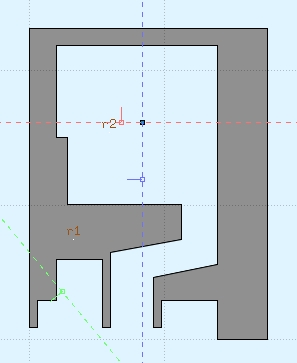# Problems with USRBIN plot

Dear experts,

As shown in the figure, it is the profile of the simulation I am doing now and the result of USRBIN.1.Why does the plot result of USRBIN was widen? How should I solve this problem?

2.The lines of the original structure can’t be seen in USRBIN plot. are there any settings in the software that can make the lines of the original structure clearer?

3.How can I get the calculation result of the specified position in USRBIN plot?

Dear @Junjie_Zhang,

I believe that you are doing the plot on a different plane than the one you are looking at in the geometry tab. In the plot tab, you need to select the plane (x, y, or z) you want to look at. Beware, that the plots in the plot tab are done using gnuplot, therefore, the ratio between the two axes (and therefore the plot) might be distorted. Beside this appearance quirk, the plot corresponds of course to the results of your simulations.
As a very interesting alternative, you can superimpose the USRBIN results directly on the geometry editor. In this case the geometry won’t be distorted.
To find out more details on how to plot (on either tab), you can have a look at slides 46 to 62 of this presentation: